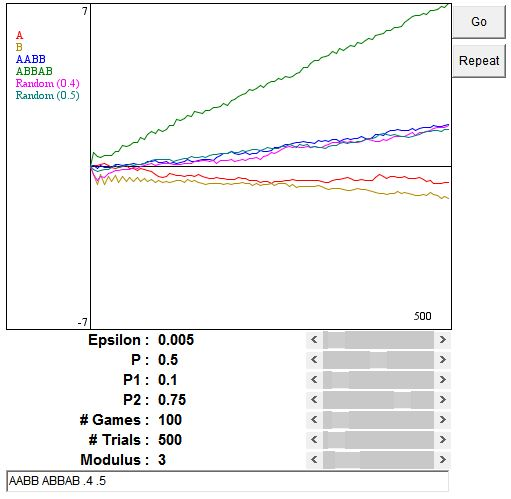For a write-up on Parrondo Paradox please see a separate page. It appears possible to combine two losing games into a winning one.

Of the two losing games -- A and B -- the first one is simple, the other one is complicated. In simple game A, one wins or loses \$1 with probabilities p and 1-p, respectively. Game B is itself a combination of two games, say B1 and B2, both being as simple as game A. In game B1 probability of winning \$1 is p1, in B2 it's p2. In B, game B1 is played if the current capital is a multiple of integer M > 1, B2 is played otherwise.

The catch here is that, in order for the paradox to occur, all three games A, B1, and B2 can't be losing. A typical assignment of probabilities would be p = .495, p1 = .095, and p2 = .745, which makes B2 a winning game. For M = 2 or 3, B still comes out a losing game, although it is winning for M > 3.

(As in the original paper by D. Abbott and G. Harmer, in the applet below, games A, B1, B2 are won with probabilities p - Epsilon, p1 - Epsilon, and p2 - Epsilon, where Epsilon is a small number around .005, but in fact may as well be zero.)

Games A and B may be combined in many different ways. They can be combined randomly with a prescribed probability of selecting, say A. Or, their selection may follow a periodic pattern, like AABB, which means deterministicly playing two A games, followed by two B games, followed by two A's, and so on. The applet allows one to define up to 7 combinations (9 is the number of distinct colors that I clearly recognize as different in my browser. Games A and B take up two of the colors.) Just type the strings of A's and B's or real numbers (for probabilities) separated by space in the edit control at the bottom of the applet. Each trial consists of a specified number of games (100, originally), and you can also specify the number of trials (500, originally).

### If you are reading this, your browser is not set to run Java applets. Try IE11 or Safari and declare the site https://www.cut-the-knot.org as trusted in the Java setup.What if applet does not run?

You may observe that the period ABBAB is by far the best strategy for M = 3, whereas AB is unsurpassed for M = 2 and M = 4. This agrees with the results of Shalosh B. Ekhad and D. Zeilberger. The former carries a Maple package PARRONDO, that, among other things, helps establish these results quite accurately. For example, for M = 3 and the probabilities defined above random strategy is optimized when A is selected with probability .4145. However, even in that case, random selection trails the periodic strategy ABBAB by a factor of about 3.

### On the Web

1. Brownian Motor, Franz-Josef Elmer, University of Basel (Java simulation)
2. J. Havil, Nonplussed!, Princeton University Press, 2007
3. Internet Bibliography of the Paradox by the inventor, Juan Manuel Rodriguez Parrondo
4. Lee Spector's CGI Simulation (Hampshire College)
5. Paradox in Game Theory: Losing Strategy That Wins, Sandra Blakeslee, The NY Times On the Web